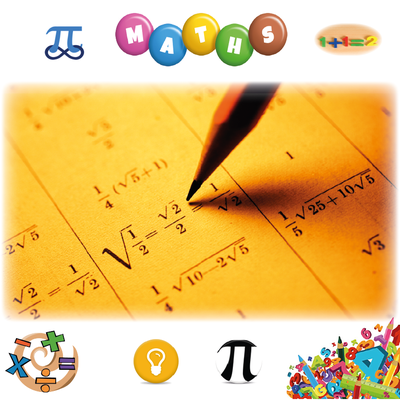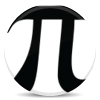## Learn ﻿﻿﻿Mathematics

An easy way to learn Mathematics online for free. Learn Maths Basics & Prealgebra; Geometry, Algebra & Trigonometry; Precalculus, Calculus & much more through this very simple course. Multiple Choice Tests. Thanks to Mem creators, Contributors & Users.
64.6k öğrenci 209h ortalama süreMaths

### 100 x 100  Multiplication Table

30.1k 81hMaths

### Maths GCSE

16.5k 50mMaths

### Learn Times Tables

16.1k 1hMaths

### Multiplication Tables

13.6k 2hMaths

### Learn ﻿﻿﻿﻿﻿﻿﻿Statistics

12k 17hMaths

### 'Basic Trigonometric Identities'

11.9k 35mMaths

### Probability Theory and Statistics (Introduction)

6.76k 7hMaths

### A level Edexcel Maths and Further Maths

6.16k 2hMaths

### 100 Perfect Squares

6.12k 2h### 150 Digits of Pi

5.42k 37mMaths

### Edexcel D1 Definitions

4.83k 25mMaths

### General Maths (Methods) CAS VCE - Trigonometry

3.73k 21mMaths

### 1 - 144 Times Tables! Multiplication and division

3.57k 5hMaths

### Conversions

3.23k 19mMaths

3.1k 22m